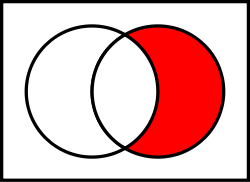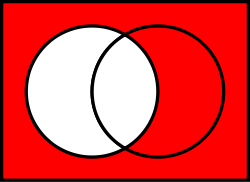# Complement (set theory)

Complement (set theory)

In set theory, a complement of a set A refers to things not in (that is, things outside of), A. The relative complement of A with respect to a set B, is the set of elements in B but not in A. When all sets under consideration are considered to be subsets of a given set U, the absolute complement of A is the set of all elements in U but not in A.

## Relative complement

If A and B are sets, then the relative complement of A in B, also known as the set-theoretic difference of B and A, is the set of elements in B, but not in A.The relative complement of A (left circle) in B (right circle):$A^c \cap B~~~~=~~~~B \setminus A$

The relative complement of A in B is denoted B ∖ A according to the ISO 31-11 standard (sometimes written B − A, but this notation is ambiguous, as in some contexts it can be interpreted as the set of all b − a, where b is taken from B and a from A).

Formally$B \setminus A = \{ x\in B \, | \, x \notin A \}.$

Examples:

• {1,2,3} ∖ {2,3,4}   =   {1}
• {2,3,4} ∖ {1,2,3}   =   {4}
• If$\mathbb{R}$ is the set of real numbers and$\mathbb{Q}$ is the set of rational numbers, then$\mathbb{R}\setminus\mathbb{Q} = \mathbb{J}$ is the set of irrational numbers.

The following lists some notable properties of relative complements in relation to the set-theoretic operations of union and intersection.

If A, B, and C are sets, then the following identities hold:

• C ∖ (A ∩ B)  =  (C ∖ A)∪(C ∖ B)
• C ∖ (A ∪ B)  =  (C ∖ A)∩(C ∖ B)
• C ∖ (B ∖ A)  =  (A ∩ C)∪(C ∖ B)
• (B ∖ A) ∩ C  =  (B ∩ C) ∖ A  =  B∩(C ∖ A)
• (B ∖ A) ∪ C  =  (B ∪ C) ∖ (A ∖ C)
• A ∖ A  =  Ø
• Ø ∖ A  =  Ø
• A ∖ Ø  =  A

## Absolute complementThe complement of A in U:$A^c=U \setminus A$

If a universe U is defined, then the relative complement of A in U is called the absolute complement (or simply complement) of A, and is denoted by Ac or sometimes A′, also the same set often is denoted by$\complement_U A$ or$\complement A$ if U is fixed, that is:

Ac  = U ∖ A.

For example, if the universe is the set of integers, then the complement of the set of odd numbers is the set of even numbers.

The following lists some important properties of absolute complements in relation to the set-theoretic operations of union and intersection.

If A and B are subsets of a universe U, then the following identities hold:

De Morgan's laws:
•$\left(A \cup B \right)^{c}=A^{c} \cap B^{c} .$
•$\left(A \cap B \right)^{c}=A^{c} \cup B^{c} .$
Complement laws:
•$A \cup A^{c} =U .$
•$A \cap A^{c} =\empty .$
•$\empty ^{c} =U.$
•$U^{c} =\empty.$
•$\text{If }A\subset B\text{, then }B^{c}\subset A^{c}.$
(this follows from the equivalence of a conditional with its contrapositive)
Involution or double complement law:
•$\left(A^{c}\right)^{c}=A.$
Relationships between relative and absolute complements:
• A ∖ B = A ∩ Bc
• (A ∖ B)c = Ac ∪ B

The first two complement laws above shows that if A is a non-empty, proper subset of U, then {A, Ac} is a partition of U.

## Notation

In the LaTeX typesetting language, the command \setminus is usually used for rendering a set difference symbol, which is similar to a backslash symbol. When rendered the \setminus command looks identical to \backslash except that it has a little more space in front and behind the slash, akin to the LaTeX sequence \mathbin{\backslash}. A variant \smallsetminus is available in the amssymb package.

## Complements in various programming languages

Some programming languages allow for manipulation of sets as data structures, using these operators or functions to construct the difference of sets a and b:

SQL
SELECT * FROM B LEFT OUTER JOIN A ON B.COLUMN = A.COLUMN WHERE A.ID IS NULL
Mathematica
Complement
MATLAB
setdiff
MathML
<apply xmlns="http://www.w3.org/1998/Math/MathML"> <setdiff/> <ci type="set">A</ci> <ci type="set">B</ci></apply>
Pascal
SetDifference := a - b;
Python
diff = a.difference(b)
diff = a - b
Java
diff = a.clone();
diff.removeAll(b);
Scala
diff = a -- b
C++
set_difference(a.begin(), a.end(), b.begin(), b.end(), result.begin());
.NET Framework
a.Except(b);
a \\ b 
Common Lisp
set-difference, nset-difference
OCaml
Set.S.diff
Unix shell
comm -23 a b
PHP
array_diff($a,$b);
R
setdiff
Ruby
diff = a - b
Perl
#for perl version >= 5.10
@a = grep {not \$_ ~~ @b} @a;

Wikimedia Foundation. 2010.

### Look at other dictionaries:

• Union (set theory) — Union of two sets …   Wikipedia

• Set theory — This article is about the branch of mathematics. For musical set theory, see Set theory (music). A Venn diagram illustrating the intersection of two sets. Set theory is the branch of mathematics that studies sets, which are collections of objects …   Wikipedia

• set theory — the branch of mathematics that deals with relations between sets. [1940 45] * * * Branch of mathematics that deals with the properties of sets. It is most valuable as applied to other areas of mathematics, which borrow from and adapt its… …   Universalium

• Set theory (music) — Example of Z relation on two pitch sets analyzable as or derivable from Z17 (Schuijer 2008, p.99), with intervals between pitch classes labeled for ease of comparison between the two sets and their common interval vector, 212320. Musical set… …   Wikipedia

• List of set theory topics — Logic portal Set theory portal …   Wikipedia

• Naive set theory — This article is about the mathematical topic. For the book of the same name, see Naive Set Theory (book). Naive set theory is one of several theories of sets used in the discussion of the foundations of mathematics. The informal content of… …   Wikipedia

• Descriptive set theory — In mathematical logic, descriptive set theory is the study of certain classes of well behaved subsets of the real line and other Polish spaces. As well as being one of the primary areas of research in set theory, it has applications to other… …   Wikipedia

• Intersection (set theory) — Intersections of the Greek, Latin and Russian alphabet (upper case graphemes) (The intersection of Greek and Latin letters is used for the Greek licence plates.) …   Wikipedia

• Ideal (set theory) — In the mathematical field of set theory, an ideal is a collection of sets that are considered to be small or negligible . Every subset of an element of the ideal must also be in the ideal (this codifies the idea that an ideal is a notion of… …   Wikipedia

• Von Neumann–Bernays–Gödel set theory — In the foundations of mathematics, von Neumann–Bernays–Gödel set theory (NBG) is an axiomatic set theory that is a conservative extension of the canonical axiomatic set theory ZFC. A statement in the language of ZFC is provable in NBG if and only …   Wikipedia